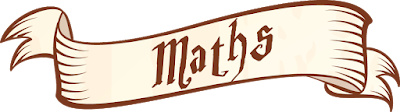## Pages

### MathsUNIT 1            NATURAL NUMBERS

UNIT 2            MULTIPLES AND DIVISORS

UNIT 3            POWERS AND SQUARE ROOTS

UNIT 4            FRACTIONS
Reducing to Common Denominator
Dividing fractions

UNIT 5            DECIMAL NUMBERS
Decimal Numbers
Rounding. decimal numbers
Adding and subtracting decimals
Multiplying decimals

UNIT 6            PERCENTAGES AND PROPORTIONALITY

UNIT 7            WHOLE NUMBERS
Comparing integers

UNIT 8            STATISTICS AND PROBABILITY

UNIT 9            LINEAR MEASURES
Units of measurement: length
Units of measurement: mass
Units of measurement: capacity
Other ways of expressing measurement

UNIT 10         NON-LINEAR MEASURES
Measuring areas
Units of measurement: area
Measuring volume
Units of measurement: volume
Relationship between capacity and volume

UNIT 11         ANGLES AND POLYGONS
Area of a circle.

UNIT 12         POLYHEDRA
Pyramids
Non-polyhedric solids
Nets and construction of polyhedra

1.2.